Compactness, countable

The property of a topological space that every infinite subset of it has an accumulation point. For a metric space the notion of countable compactness is the same as that of compactness. The property of countable compactness can be expressed in the following form: Every countable subset has an accumulation point, so that countably compact spaces are naturally called-compact.

In connection with this arise the concepts of initial and final compactness, or more generally compactness in an interval of cardinals, or-compactness, expressible in three equivalent forms: 1) each set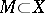of cardinality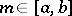has a complete accumulation point, that is, a pointsuch that for every neighbourhood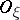of it, the set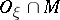has the same cardinality as; 2) every totally ordered system of order typeof closed sets has a non-empty intersection; and 3) every open covering of cardinalitycontains a subcovering of cardinality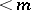.

Ifequals, thenis called initially compact up to cardinality. Countable compactness means initial compactness up to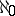. If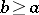is arbitrary, thenis called finally compact, starting from cardinality; thus, every space with a countable basis is finally compact from. Compact spaces are initially compact from any (infinite) cardinality and are at the same time finally compact starting from any cardinality. Thus, every compact space is countably compact, but not conversely: The space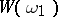of all ordinal numbersis countably compact, but not compact. The (countable) compactness of a spaceneed not imply that it is sequentially compact. E.g., in the (non-metrizable) spacethere exists an infinite closed (and hence Hausdorff compact) set containing no non-stationary convergent subsequence. (Cf. Compact set, countably.)

In particular, the property that each infinite set has an accumulation point (or limit point of a set, which is the same thing) is equivalent to the property of being countably compact as defined in the article Compact space, i.e. in the sense of the property that each countable covering has a finite subcovering. A space is a compact if each infinite subset has a complete accumulation point (cf. [a1]). Finally compact (from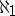) spaces are called Lindelöf spaces in Western terminology.
In the article above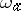is the first ordinal number of cardinality, in standard Western usage, however,is the-th infinite cardinal number.